Updated date:

# Using the Minkowski Diagram

Author:

## Contents

How both the observer and the object see a linear contraction of the other

How the observer and the object measure time dilation in other system

The speed of light as measured in both systems

Each coordinate system sees a lack of simultaneity in the other coordinate system

An electromagnetic wave within the observer’s system

An electromagnetic wave within the moving object’s system

The Doppler effects on electromagnetic waves from a moving source

The Addition of Velocities on the Diagram

Using the Minkowski diagram to show relationship between mass and momentum

Using the Minkowski diagram to show relationship between momentum and energy

The invariance of like space-time areas and volumes

The Minkowski diagram offers a way to visualize and to answer some questions about the special theory of relativity. It can also be used to show the relationship between mass & momentum and energy & momrntum.

## How both the observer and the object see a linear contraction of the other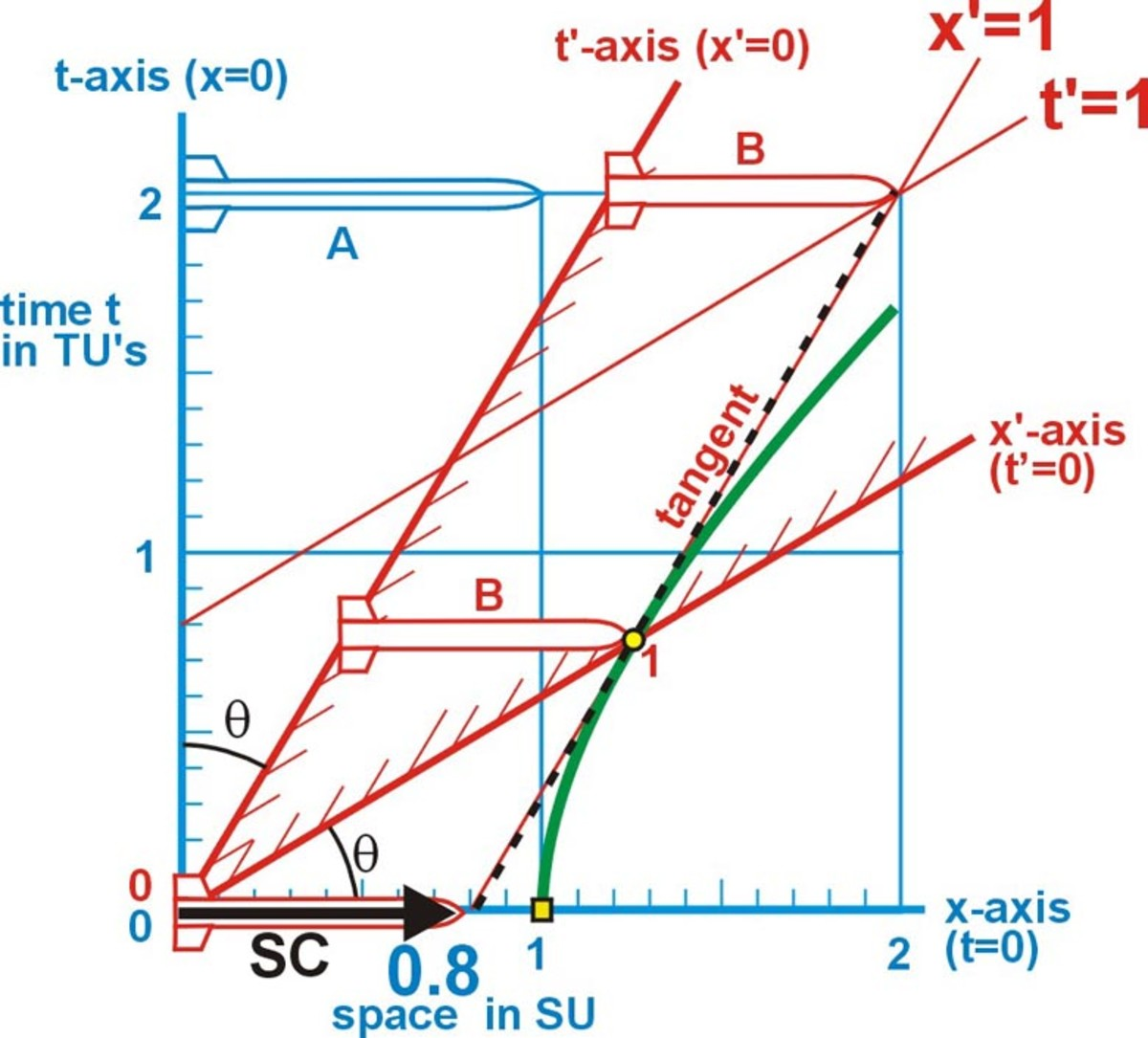Fig. 1 Spatial contraction of object

When there is no movement between the object's rocket (B in red) and the observer's rocket (A in blue) they are both one unit in length. In fig 1 the object is passing the observer with a speed of 0.6c. The observer measures that the object's rocket (in red) has contracted to 1/g = 0.8 its original length. The relativity factor g (gamma) = 1/(1-v2/c2) ½ .The observer's rocket (blue) is one unit long on any of its lines of simultaneity (any horizontal line). The object's rocket (red) is 0.8 units long on any of the observer's lines of simultaneity and shown at several different times. This illustrates the spatial contraction (the SC arrow).

Draw a tangent (dotted black line) to the space hyperbola at the point where the object's spatial axis (x') crosses the hyperbola. The distance from the origin to where this tangent crosses the observer's spatial axis (x) is equal to the spatial contraction.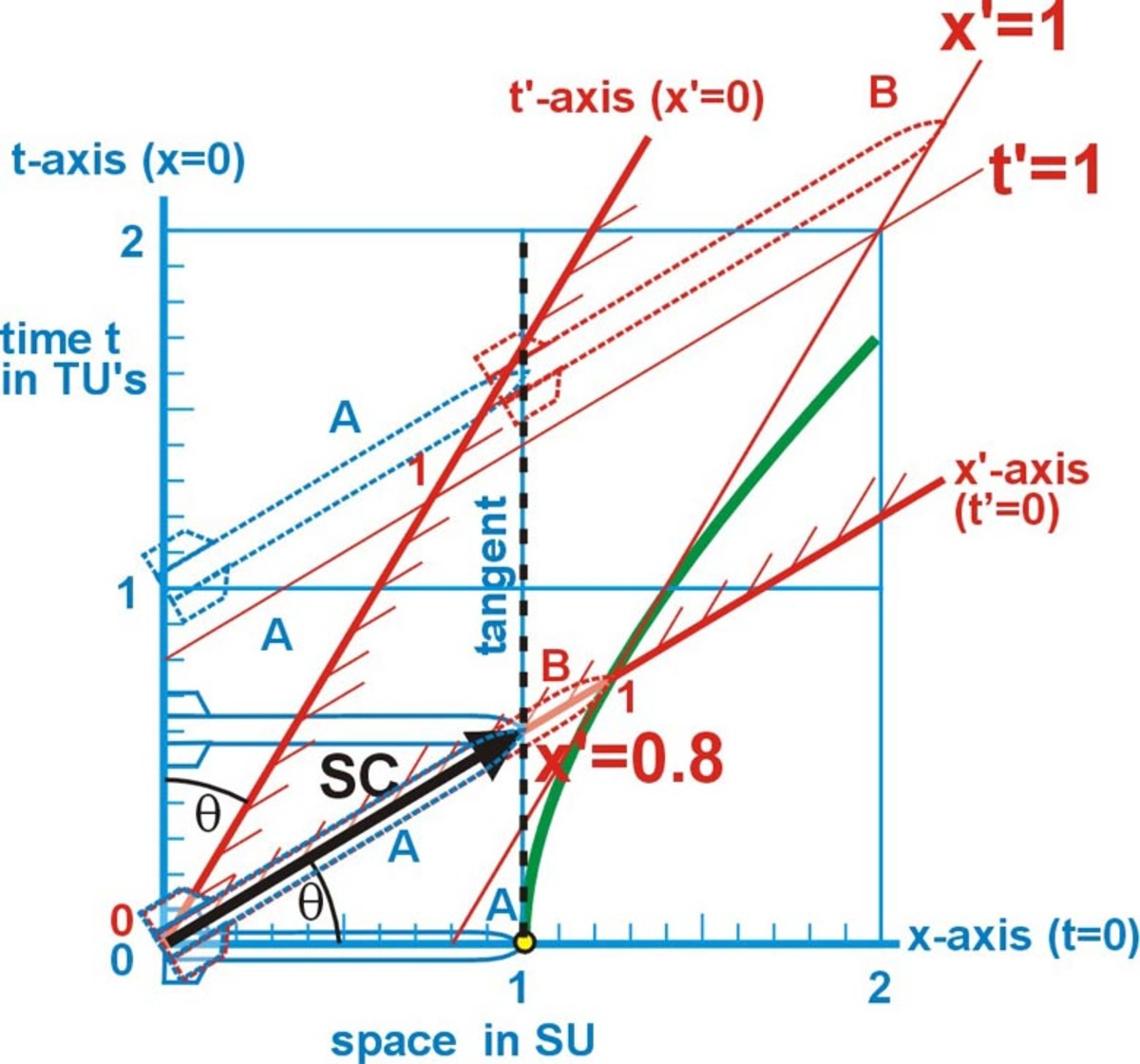Fig. 2 Spatial contraction of observer

In fig 2 an observer on rocket B (the object's rocket) will measure B's length (in red) along any of its lines of simultaneity (time lines) as 1 SU in length. From x' = 0 to x' = 1. In B's system any line that is parallel to B's spatial axis (x'-axis) is a line of simultaneity. B will measure A's length (in blue) as 0.8 SU. The distance from the observer's t-axis to its x = 1 line is 0.8 units along any of B's lines of simultaneity. Therefore B sees the same spatial contraction (the SC arrow) in A as A saw in B.

Draw a tangent (dotted black line) to the space hyperbola at the point where the observer's spatial axis (x) crosses the hyperbola. The distance from the origin to where this tangent crosses the object's spatial axis (x') is equal to the observer's spatial contraction (0.8 units) as seen by observer B on the object.

In both cases the contraction is illustrated on the space axis between the space lines.

## How the observer and the object measure time dilation in other system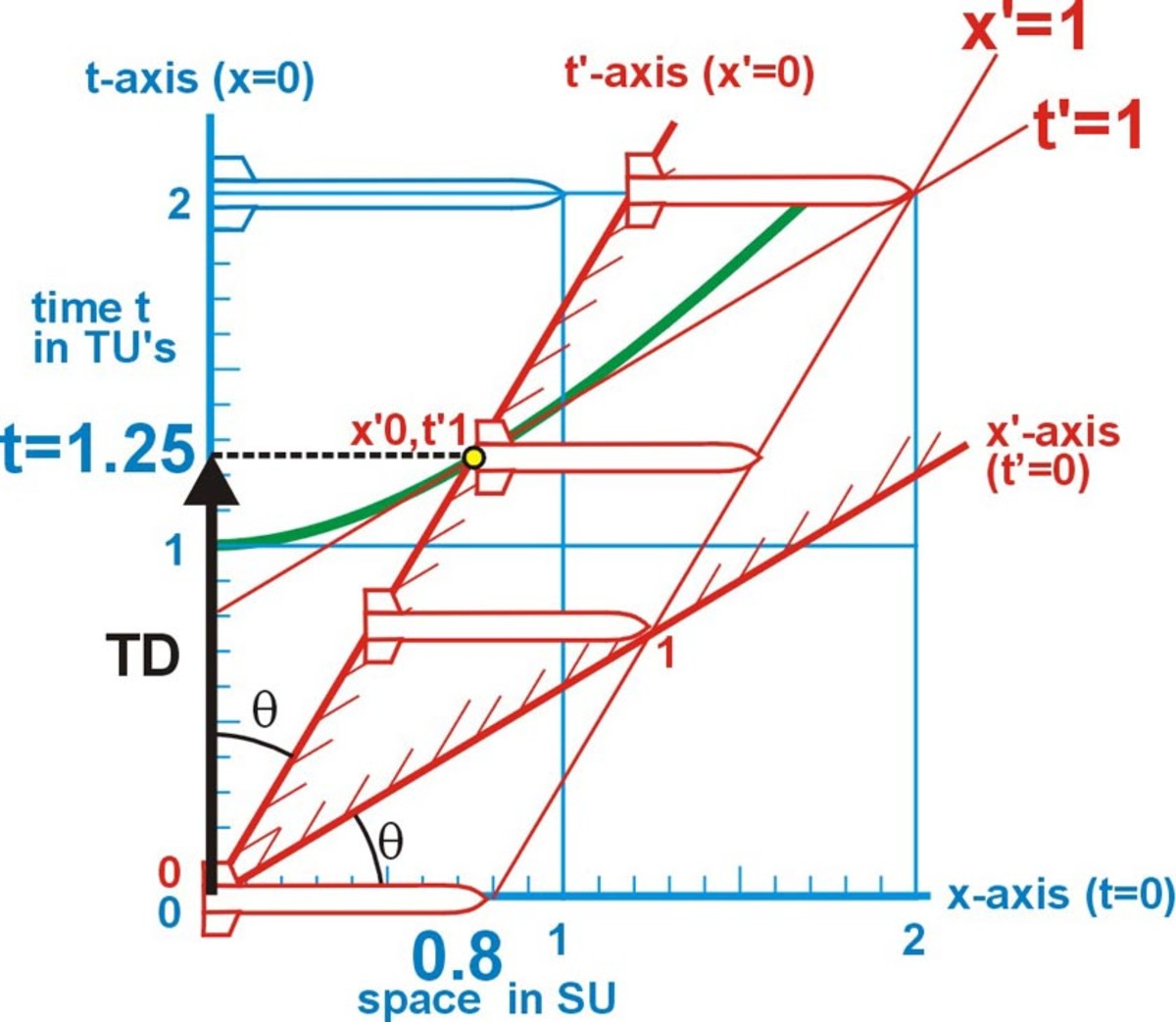Fig. 3 Time dilation in object

In fig. 3 the object is passing the observer with a speed of 0.6. By the time the object's rocket has traveled for one of its time units, 1.25 time units have passed in the observers system. That is, when the observer's line of simultaneity (dotted black line) passes through the object's one time unit, it is at the observer's 1.25 time unit. This illustrates the time dilation g (the TD arrow on the observer's time axis). Time is moving slower in the object's system than in the observers system.

The distance from the origin to the observer's line of simultaneity, that passes through the intersection

of the object's time axis (t') with the time hyperbola, is equal to the time dilation (1.25 TU).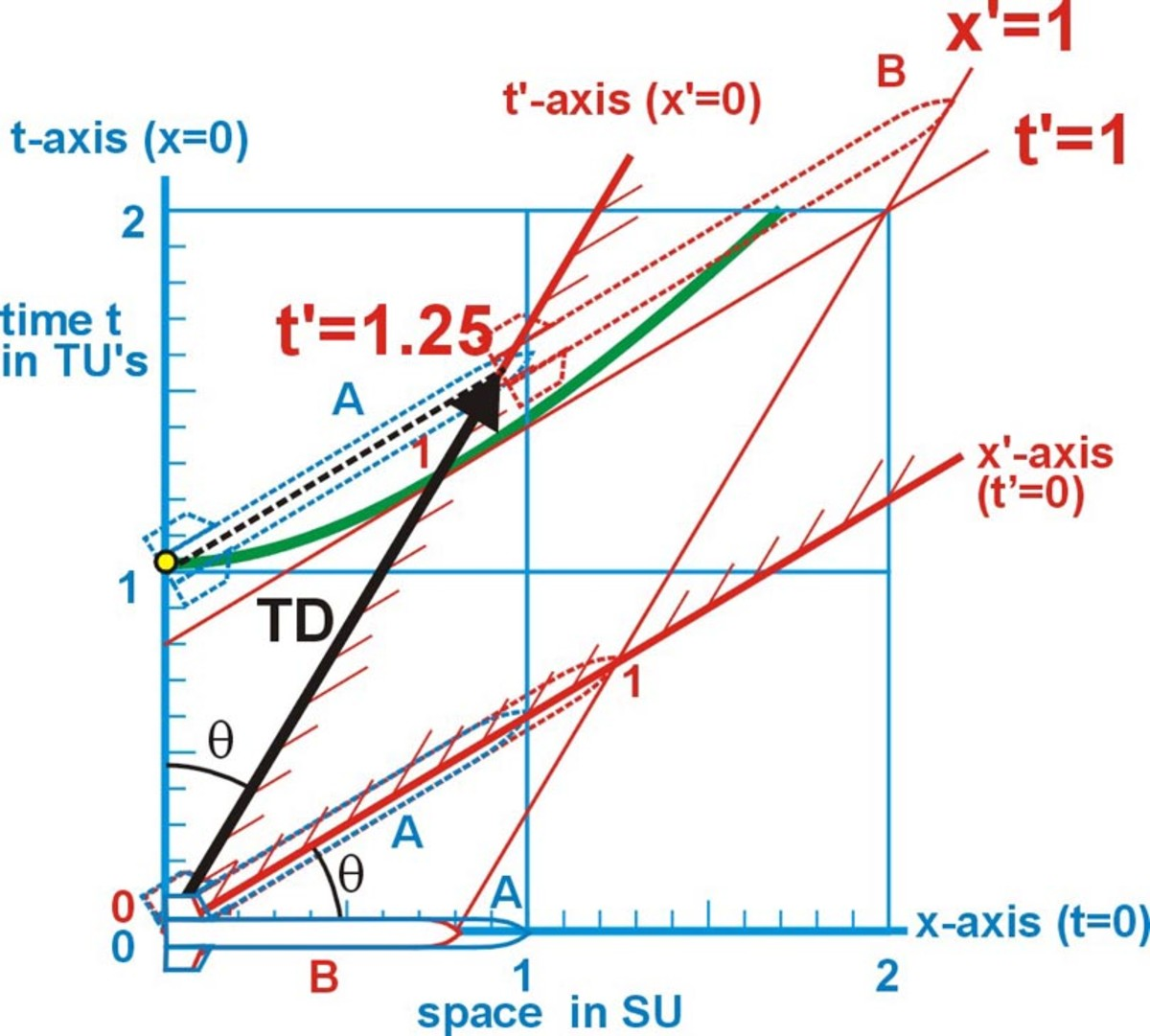Fig. 4 Time dilation in observer

In fig. 4 by the time the observer's rocket (A) has passed through one of its time units, 1.25 time units have passed in the object's system (B). That is, when the object's line of simultaneity (dotted black line) passes through the observer's one time unit, it is at the object's 1.25 time unit. This illustrates the time dilation g (the TD arrow on the object's time axis). B sees time moving slower on A than on B. Here the rockets are shown on both A's and B's lines of simultaneity for comparison. This illustrates how both the observer and the object measure the same time dilation in the other system.

The distance from the origin, along the object's time axis, to the object's line of simultaneity, that passes through the intersection of observer's time axis (t) with the time hyperbola, is equal to the time dilation (1.25 T'U).

## The speed of light as measured in both systems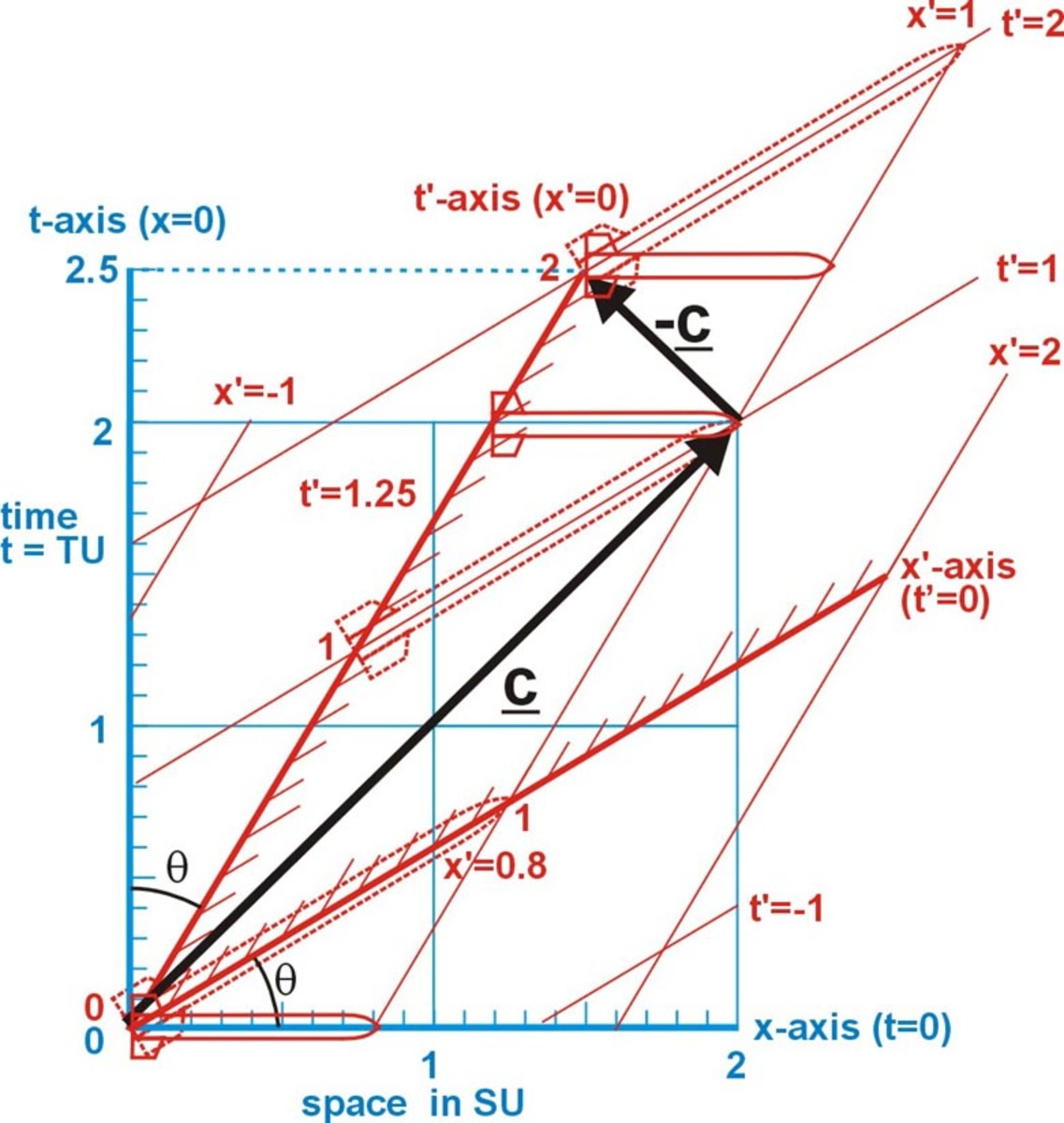Fig. 5 The speed of light to object

In fig. 5 the observer sees the object as moving forward (to the right) at a relative speed of 0.6c. The object measures that it takes 1 time (t'=1) unit for light to travel from the back of his rocket to the front. However, the observer will measure that the event took 2.0 (t=2) of the observer's time units. The object measures that it takes 1 time unit for light to travel from the front of his rocket to the back. The observer will measure the event took only 0.5 time units. Thus the object measures that it takes 2 time units for light to travel from the back of his rocket to the front and return to the back. However, the observer will measure that the event took (c+v)g/c + (c-v)g/c = 2g = 2(1.25) = 2.5 time units.

The object measures that it takes 1 time unit for light to travel from the back of his rocket (which is 1 space unit long) to the front. And it takes 1 time unit for light to travel from the front of his rocket to the back. Then the speed of light is the constant c = 1SU/1TU relative to him.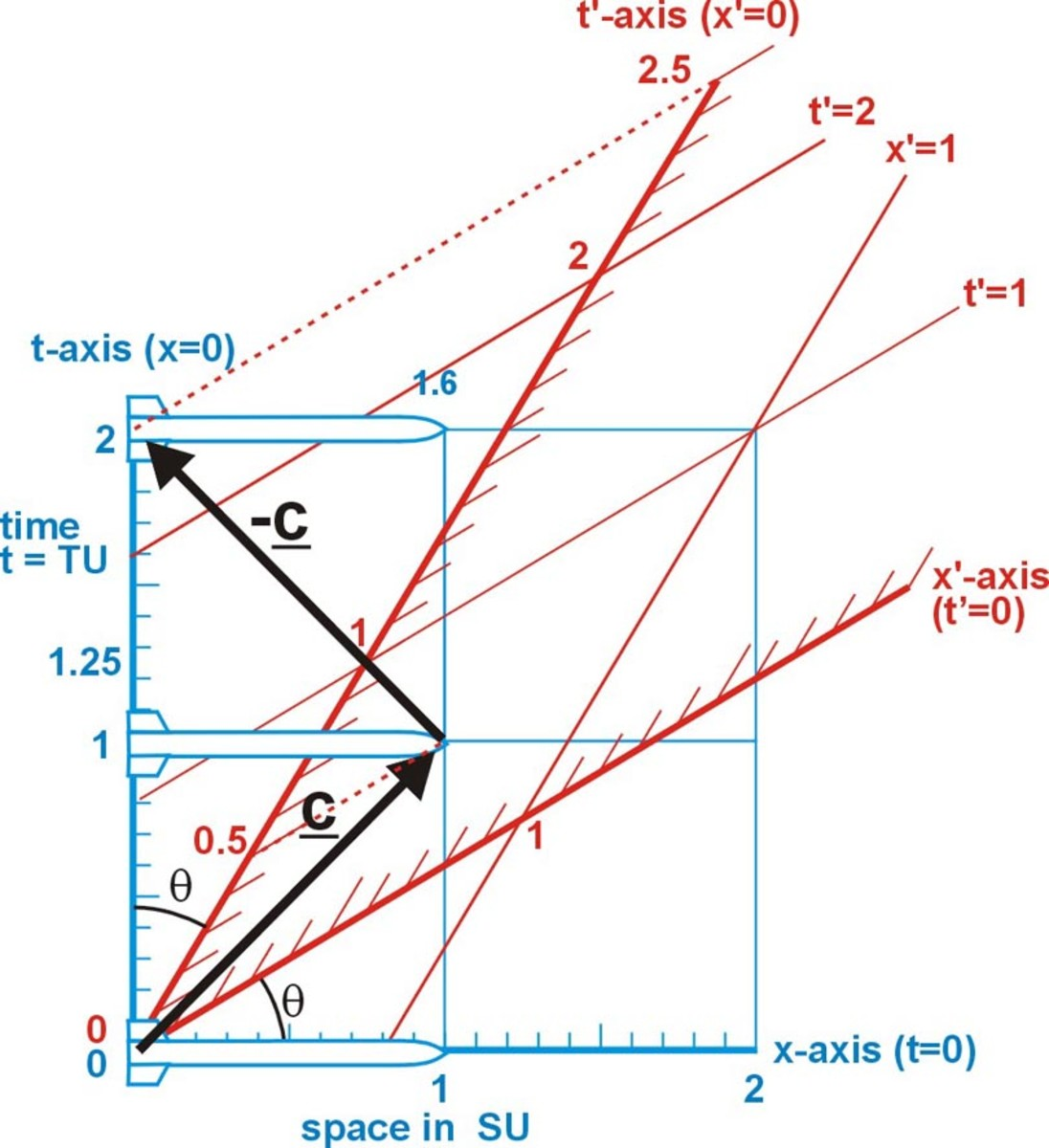Fig. 6 The speed of light to the observer

In fig. 6 the object sees the observer as moving backward (to the left) at a relative speed of 0.6c. The observer measures that it takes 1 time unit (t=1) for light to travel from the back of his rocket to the front. However, the object will measure that the event only took 0.5 time units (t'=0.5). The observer measures that it takes 1 time unit for light to travel from the front of his rocket to the back. The object will measure the event took 2.0 time units (2,5-0.5). Thus the observer measures that it takes 2 time units for light to travel from the back of his rocket to the front and return to the back. However, the object will measure that the event took 2.5 time units.

## Each coordinate system sees a lack of simultaneity in the other coordinate system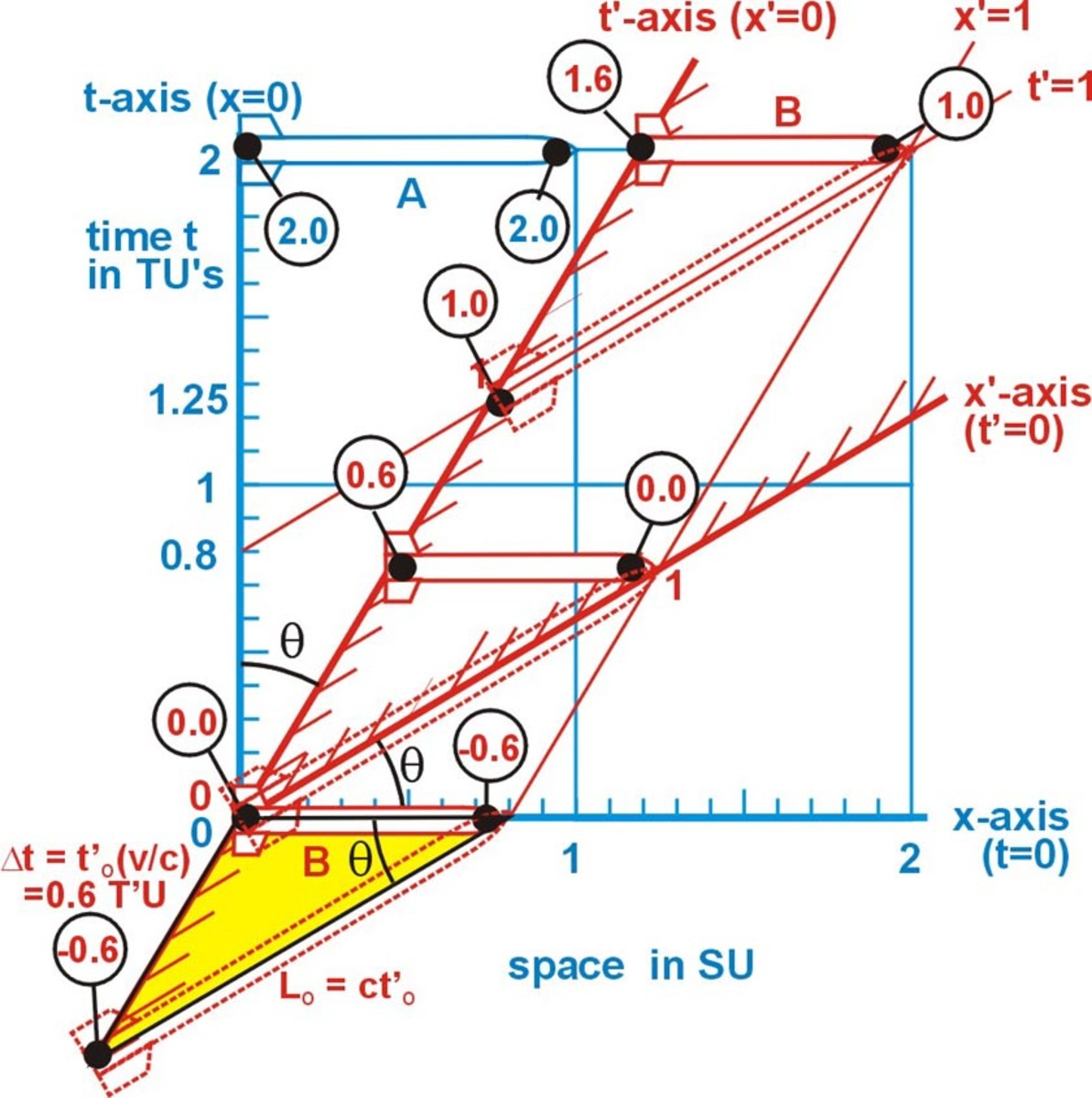Fig. 7 The observer sees a Lack of Simultaneity in object with a relative speed of 0.6c

In fig. 7 the circles represent clock readings at the front and rear of the rocket. The object B (in red) is shone on his own time line and on the observer's time line at several different time intervals. The observer A (in blue) will see his own clocks as being synchronized, both the front and rear clocks read the same time along his line of simultaneity (shown here at t = 2). At the observer's 0 time unit, the clock at rear of the object's rocket also reads 0. However, the clock at the front of the object's rocket reads minus 0.6 time units.

When the clock at rear of the object's rocket reads 0.6 and the clock at the front of the rocket reads 0 time units. This illustrates that the trailing clock is v/c (0.6) time units ahead of the leading clock when they are one of their space units apart (LO). LO is the distance measured by the object under observation. That is to the observer the clocks of the object are offset by LO(v/c2)** Here LO is the distance light would travel in one time unit ctO. Hence, ctO(v/c2) equals a time difference of tO(v/c).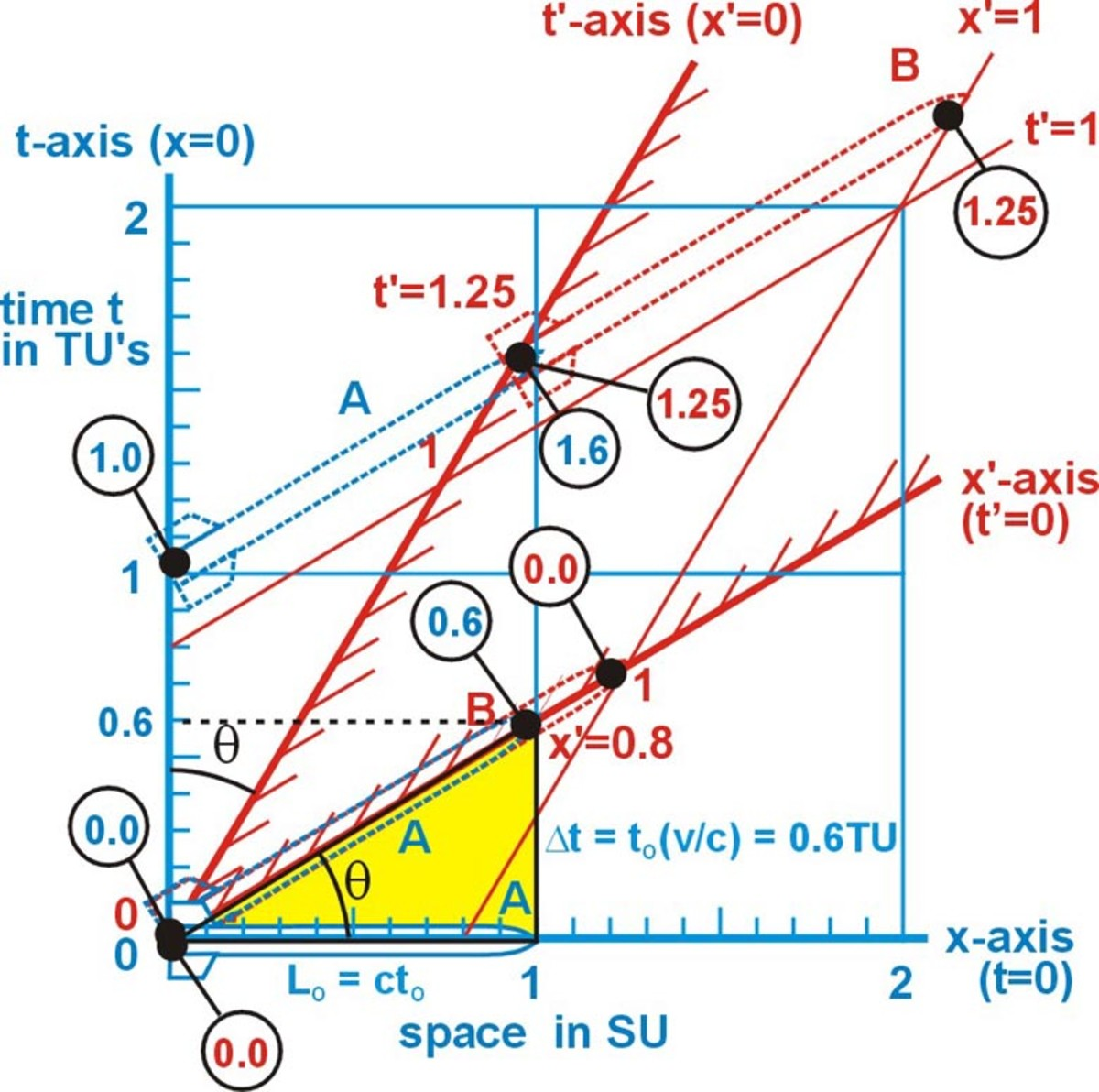Fig. 8 The object sees a Lack of Simultaneity in observer with a relative speed of 0.6c

In fig. 8 the object (B) will see his own clocks as being synchronized, both the front and rear clocks read the same time along his line of simultaneity (shown at t' = 0 and t' = 1.25). B will see the clocks at the front and back of A as offset by 0.6t. However, B sees A's forward clock as being ahead the clock at the rear of A. This is because B sees A as moving backward (to the left) away from B. Hence B sees the clocks offset on A as A saw them offset on B.

The amount of time offset between the clocks is due to the distance LO between the clocks and the angle q between the two spatial axes. The time difference Dt = tO tan q = tO(v/c). Where tO = LO/c. This is illustrated with the yellow triangles in both illustrations above. The side of the triangle opposite the angle q and parallel with the time axis, has a length equal to the time difference Dt = tO(v/c). The amount of time offset is indicated by the scale of the time axis.

**Physics1 by Elisha Huggins, Physics by Tipler

## An electromagnetic wave within the observer’s system.

In fig. 9 we see the observer's rocket is two space units long. At t = 0 an electromagnetic wave is emitted at the center of the rocket. The wave's wavelength (l) is one space unit (SU). The wave starts at

t = 0 and advances in both directions with a velocity of c. At t = 0.5 the wave has traveled ½ wavelength in both directions. After one time unit the wave has traveled one wavelength to the right and one wavelength to the left. The wave has arrived at both the front and back of the rocket at that instant. The period (T) of the wave (the time to complete one cycle) is one time unit (1TU) here. The frequency (f or n) of the wave (the number of cycles to past the observer in one time unit) is one cycle per time unit.

Even though frequency is not a space unit it can be indicated as a distance on the observer's space scale by equating the number of wavelengths that will pass the observer in one time unit. This frequency can be indicated on the diagram as the horizontal distance from the end of one complete wavelength to the observer's time axis. The frequency on the diagram can also be indicated as the vertical distance from the end of one complete wavelength to the observer's space axis.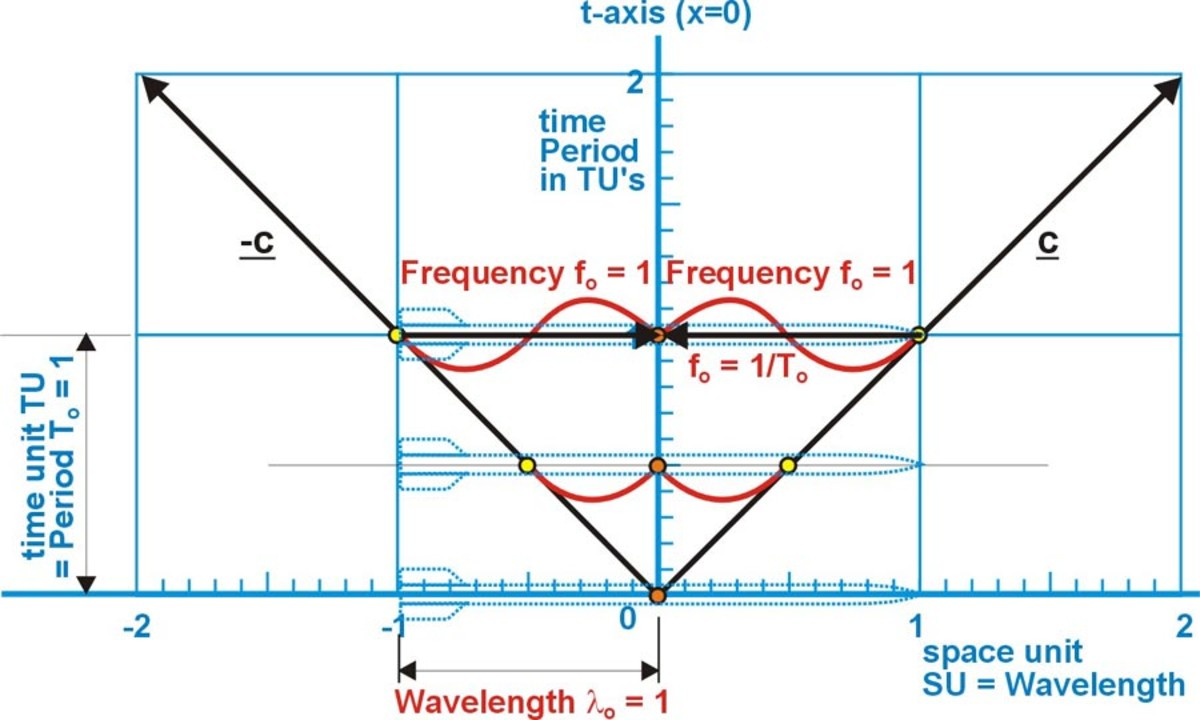Fig. 9 The diagram of an electromagnetic wave emanating from the center of the observer’s rocket.

## An electromagnetic wave within the moving object’s system.

Now let us observe from the object's view, the same wave being emitted in the moving object's system. The diagram will still be drawn from the observer's point of view. Here the object's vehicle is moving past the observer with a relative speed of 0.6c. In fig. 9 we see the object's rocket is two space units SU' long. At t = 0 an electromagnetic wave is emitted at the center of the rocket. The object sees identically the same thing with his wave as the observer saw with his wave. The object's wave has a wavelength (l') that is one space unit (SU'). The wave starts at t' = 0 and advances in both directions with a velocity of c. At t' = 0.5 the wave has traveled ½ wavelength in both directions. After one of the object's time units the wave has traveled one wavelength to the right and one wavelength to the left. The wave has arrived at both the front and back of the rocket at that instant. The time period (T') of the wave (the time to complete one cycle) is one of the object's time units (1TU'). The frequency (f') of the wave (the number of cycles in one time unit) is one cycle per one of the object's time unit. The frequency (f') of the wave can be illustrated by the number of wavelengths that pass a point in one time unit.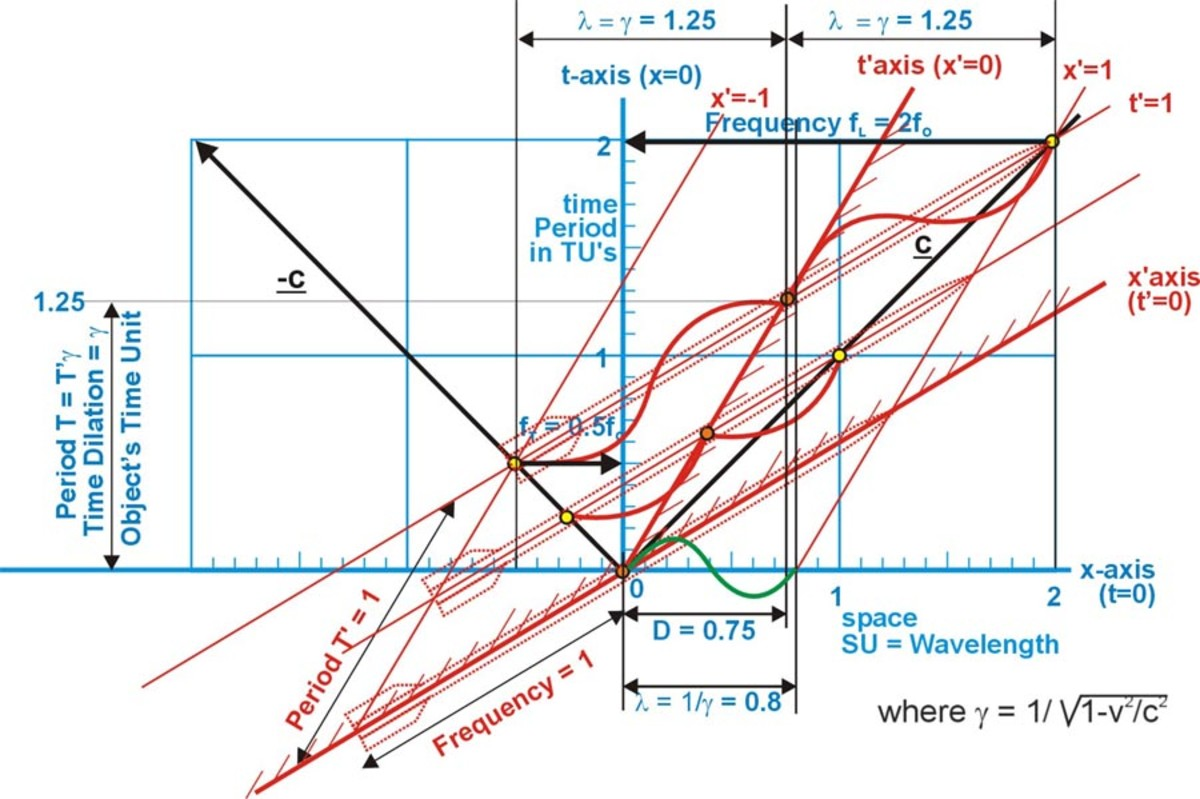Fig. 10 The effects on electromagnetic waves from the object moving with a relative speed of 0.6c to the right

The object's wavelength can appear both longer and shorter in the diagram. In fig. 10 the observer sees that it takes longer for events to occur in the object's system than for the same events to occur in his own system. Time in the object's system is dilated by g = 1.25 observer's time units. This means the time period T' of the wave, the time to generate one cycle, will now take 1.25 TU's. Since the time period is longer, the wave's wavelength l, will be stretched on the diagram to g = 1.25 of the observer's space units SU. This indicated by the arrows at the top of fig 2-14.

However, if the wave is confined to the rocket as a standing wave its wavelength will be contracted to 0.8 its original length on the observer's line of simultaneity. That is the same contraction that of the rocket. This is shown on the diagram as green wave line on the observer's space axis. Otherwise the wave will radiate away from the rocket with its wavelength affected by the Doppler effect.

## The Doppler effects on electromagnetic waves from a moving source

From the observer's viewpoint.

In figure 11 the observer sees the Doppler effect on the signal from the object. The observer sees the object moving away from him to the right, with a speed of 0.6c. The time unit of the wave is the time it takes for one complete cycle (one wavelength) to be emitted in the object's system (t'=1) is g = 1.25 time units (t=1.25) in the observer's system. An observer's line of simultaneity is drawn at this t = 1.25 time units. At this time the wave front has moved g = 1.25 space units to the right and to the left. During this time the object emitting the wave, has moved to the right the distance D = g(v/c) = 1.25(0.6) = 0.75 space units (x = 0.75).

The leading Doppler affected wave lL is compressed between the point of emission and the black line c representing the speed of light to the right. This leading wavelength is lL = g - D = 1.25 - 0.75 = 0.5 of the observer's space units and is drawn as a heavy black wave line. This length is also indicated by the top of the small yellow triangle. The time period of this wave is the time required for one wavelength to pass a given point in the observer's system. The left side of this triangle represents the period TL of this wave. The frequency (the number cycles per observer time unit) of this wave is indicated by the horizontal black arrow fL from the point (x'=1,t'=1) to the observer's time axis. This arrow is 2 space units long which would indicate a frequency of 2 cycles per time unit. This frequency is f L = g + D = 1.25 + 0.75 = 2.0 cycles per the observer's time unit. This equals the relativitic Doppler equation for leading frequency.

The trailing Doppler affected wave lT is stretched out between the point of emission and the black line -c which represents the speed of light to the left. This wavelength is 1.25 + 0.75 = 2.0 of the observer's space units. This is drawn as a heavy black wave line. This length is also indicated by the top of the large yellow triangle. The right side of this triangle represents the period TT of this wave. The frequency of this wave is indicated by the horizontal black arrow from the left side of the wave to the observer's time axis. This arrow is 0.5 time units long which would indicate a frequency of ½ cycles per time unit. This frequency is

f T = g - D = 1.25 - 0.75 = 0.5 cycles per the observer's time unit. This matches the Relativistic Doppler Effect equation* for frequency is f = fO[1-(v/c)2]1/2/[1-(v/c)cos q] and for wavelength l = lO[1-(v/c)cos q]/ [1-(v/c)2]1/2.

*Schaum's Outline Series - Modern Physics by Gautreau & Savin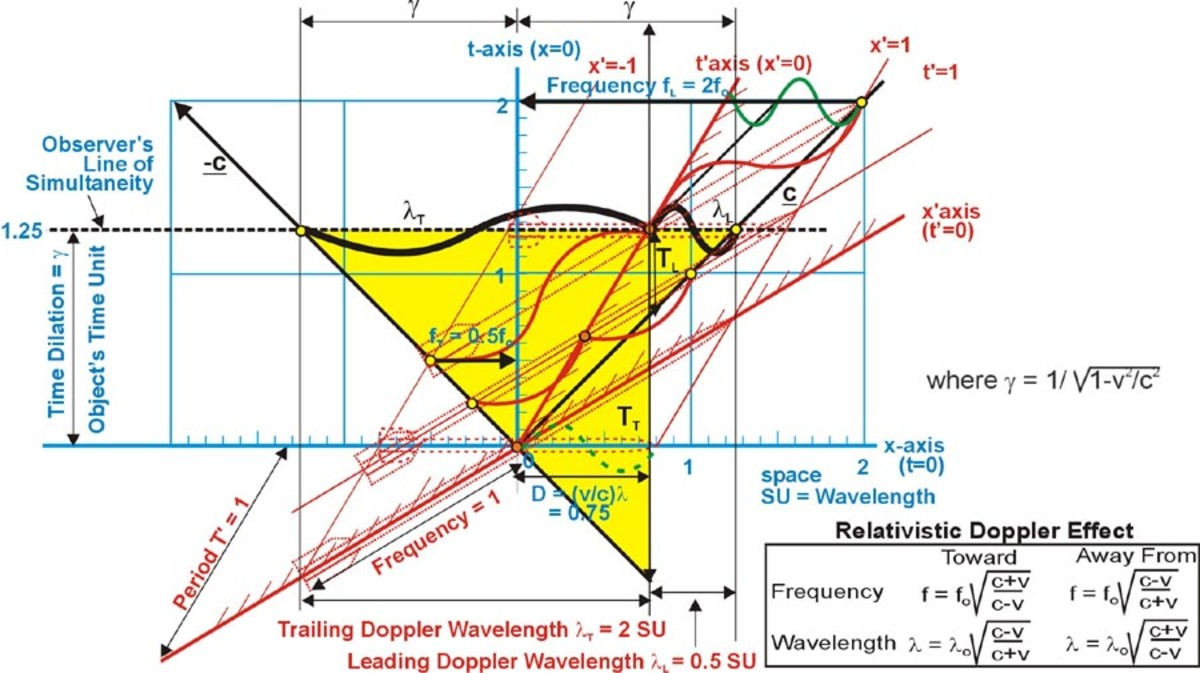Fig. 11 The Doppler effects on electromagnetic waves from an object with a relative speed of 0.6c to the observer

From the object's view point.

Figure 12 shows the same Doppler effect on the observer's system seen by the object. The leading and trailing Doppler affected waves are on the opposite sides since the observer is moving to the left from the object's view point.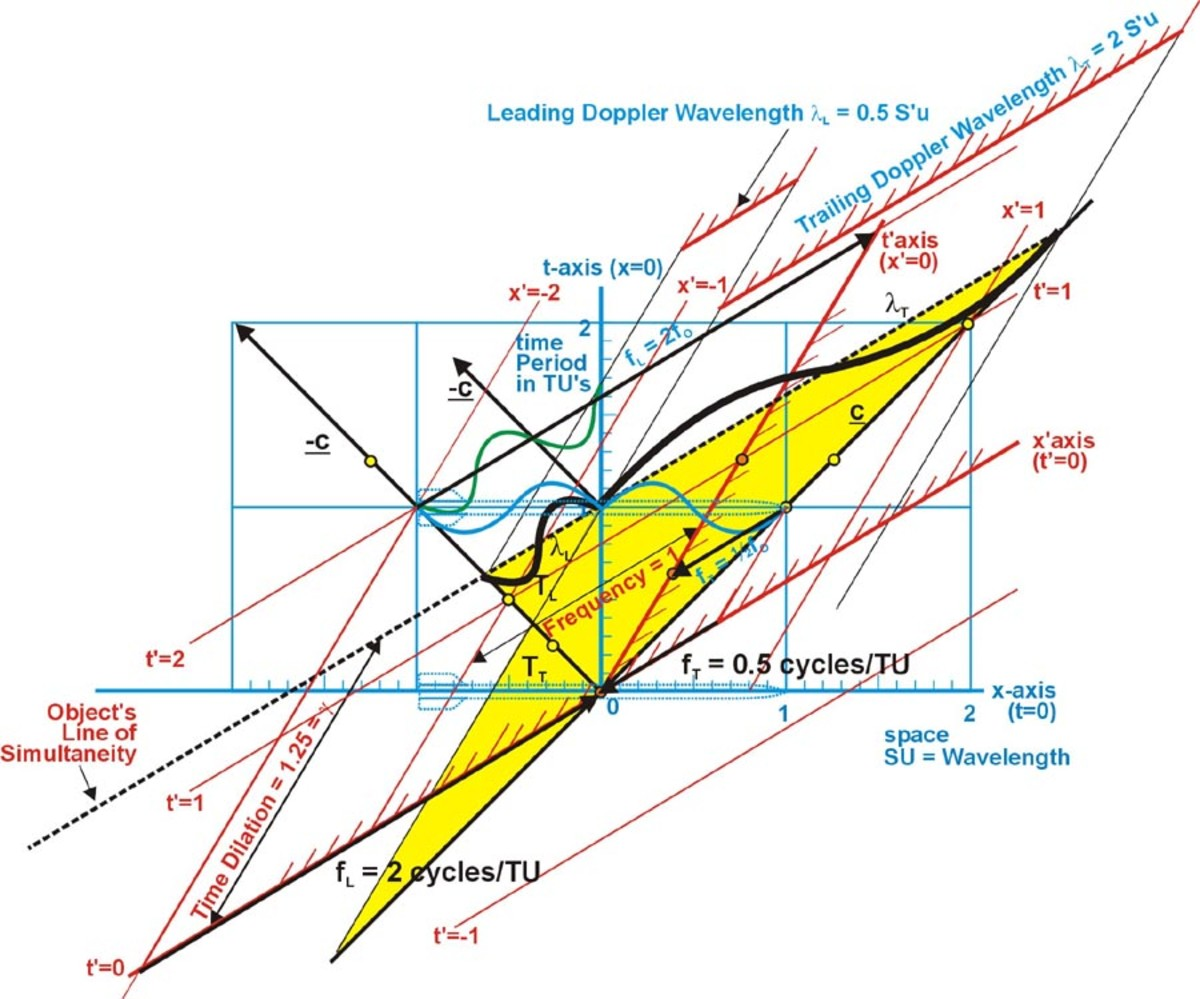## The Addition of Velocities on the Diagram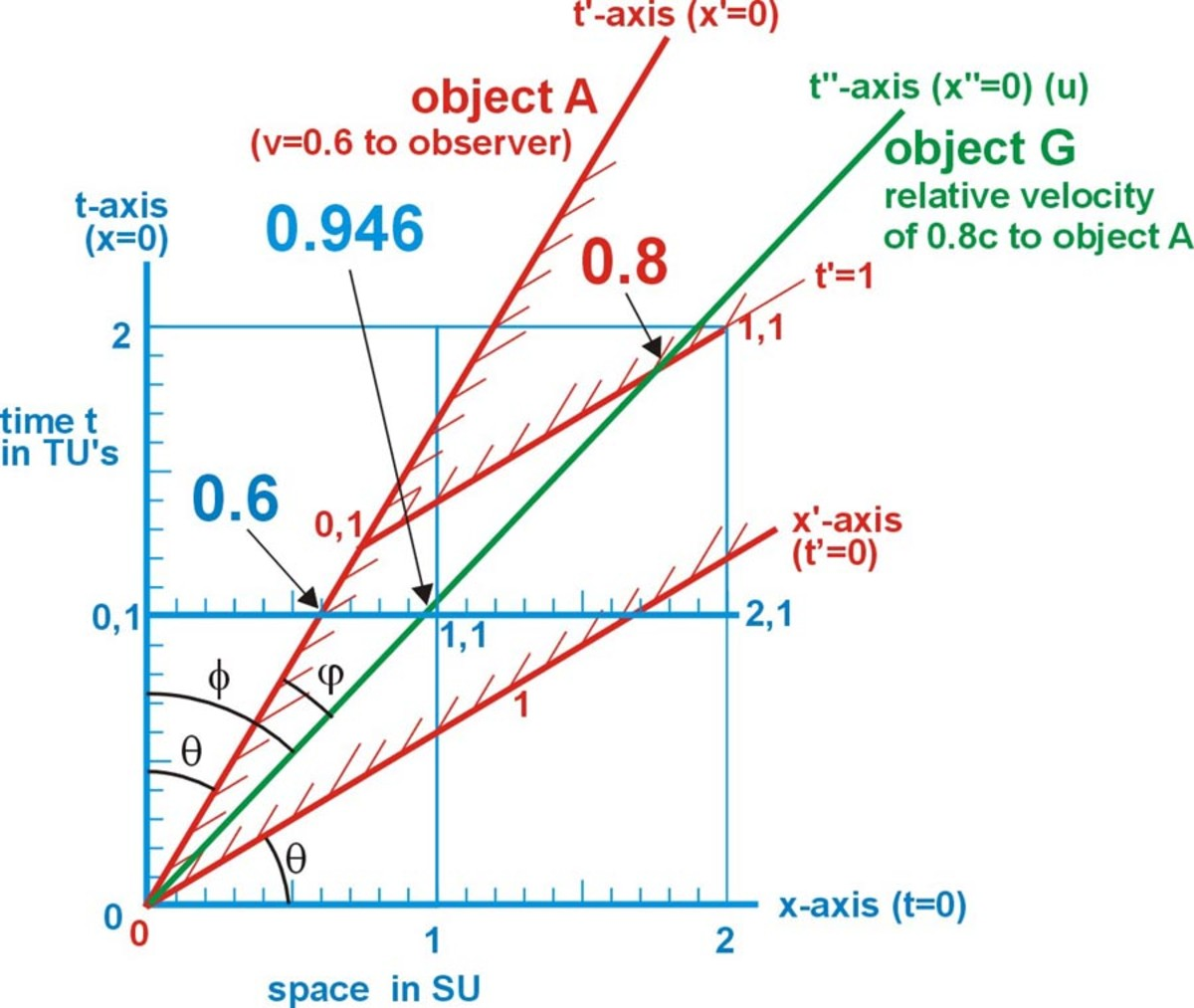Fig. 13 The addition of velocities of v = 0.6c and u = 0.8c in the same direction

In the fig 13 object A shown in red lines, is passing an observer with a relative velocity of v = 0.6c.. After one of the observer's time units has passed, the object will have moved a distance from the observer of 0.6 of the observer's space units. That is the object's path (its T'-axis) through the observer's space-time coordinates will cross the observer's one time unit at 0.6 space units.

A second object G has a relative velocity of u = 0.8c to the first object and is shown in green. The second object's path (its T"-axis) passes through the first object's one time unit line at 0.8 space units. This same green line passes through the observer's one time line at about 0.95 space units. This means the slope of the green line is about 0.95 to the observer's. Thus, the second object G must have a relative velocity to the observer of about 0.95c.

We can use the inverse Lorentz transformations to find the velocity V of the object G to the observer.

V/c = x/t = (x'+vt')/(t'+vx') = (0.8+0.6)/(1.0+0.48) = 1.4/1.48 = 0.945946 thus V = 0.945946c

We can compare this to the results using the relativistic equation for adding velocities*.

V = (v+u)/((1+vu)/c2) = 1.4c/(1.48c2/c2) = 0.945946c

These calculations could have been used to construct the above diagram. In the diagram no velocity of an object can occur in the gray shaded area.

*Modern Physics by Ronald Gautreau & William Savin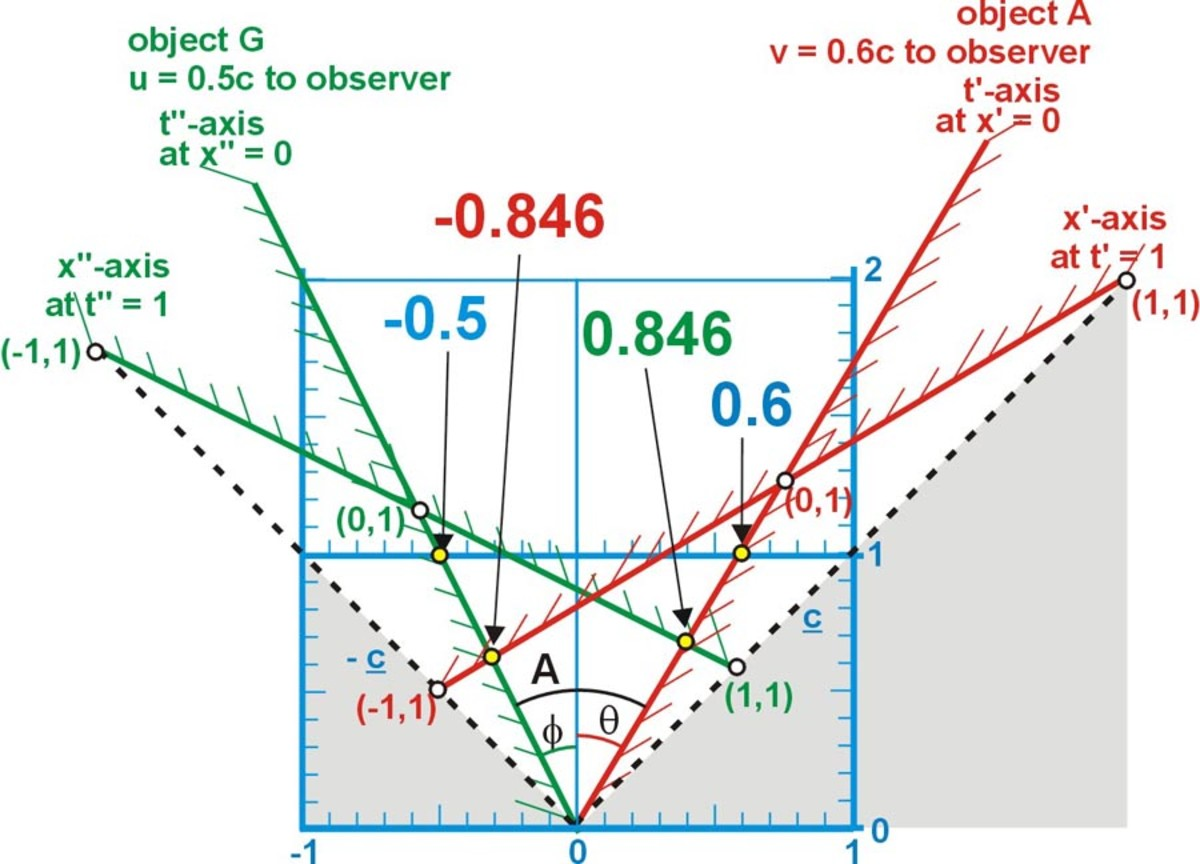Fig. 14 The addition of velocities of v = 0.6c and u = - 0.5c in the opposite directions

In fig. 14 the time-axis t' for object A (in red), has a relative velocity of 0.6c to the observer. We see that the t' axis intersects the observer's one time unit line at 0.6x, the distance that object A would travel in one of the observer's time units. We also see the time-axis t" for object G (in green), which has a relative velocity of -0.5c to the observer. We see that the t" axis intersects the observer's minus one time unit line at -0.5x, the distance that object G would travel in one of the observer's time units. The difference between these two velocities is U = v - u = 0.6c - (-0.5c) = 1.1c. However, by using the relativistic equation for adding velocities

U = (v-(-u))/((1- (-vu))/c2) = 1.1c/1.3c2/c2 = 0.8461539c**

In the diagram, we see the time axis t' of object A intersects the spatial axis x" (at t"=1) of object G at about 0.846 x" units. This is the distance that object A would travel in object G's coordinate system in one time unit.

We also see the time axis t" of object G intersects the spatial axis x' (at t'=1) of object A at about -0.846 x' units. This is the distance that object G would travel in object A's coordinate system in one time unit.

Thus, illustrating, that both object A and object G measure their relative velocity to each other as 0.846c.

We can use the Lorentz transformations to find the velocity V of the object G to the object A or the velocity U of the object A to the object G.

V/c = x"/t" = (x-vt)/(t-vx) = (-0.5-0.6)/(1.0-(-0.3)) = -1.1/1.3 = -0.8461539 thus V = -0.8461539c

U/c = x'/t' = (x-ut)/(t-ux) = (0.6-(-0.5))/(1.0-(-0.3)) = 1.1/1.3 = 0.8461539 thus U = 0.8461539c

**Physics1 by Elisha Huggins, Physics by Tipler

## Using the Minkowski diagram to show relationship between

So far we have been using the x,t Minkowski diagram to study the ratio v/c of the relative speed v of the object to the speed of light c. When both sides of a ratio are multiplied by the same number the ratio remains the same. The relationship between the mass of an object and its relative velocity to an observer is given by m = gmO, where g = 1/(1+v2/c2))1/2 and mO is the rest mass. The rest mass is the mass of that the object would have when at rest to the observer. The mass (m) and momentum (p = mv) of the object varies with its relative velocity to the observer. The mass equation is the same as the time equation by interchanging m for t. Thus we can use our existing x,t Minkowski diagram by substituting m for t and p/c = mv/c for x (where x = tv/c), to illustrate mass (m) vs. momentum (mv/c). For the mass diagram the magnitude of the mass M of the object particle plotted relative to particle's momentum divided by c;

MO2 = m2 -(px/c) 2-(py/c) 2-(pz/c) 2 = M'O2 = m'2-(px'/c) 2-(py'/c) 2-(pz'/c) 2 is invariant of all inertial frames.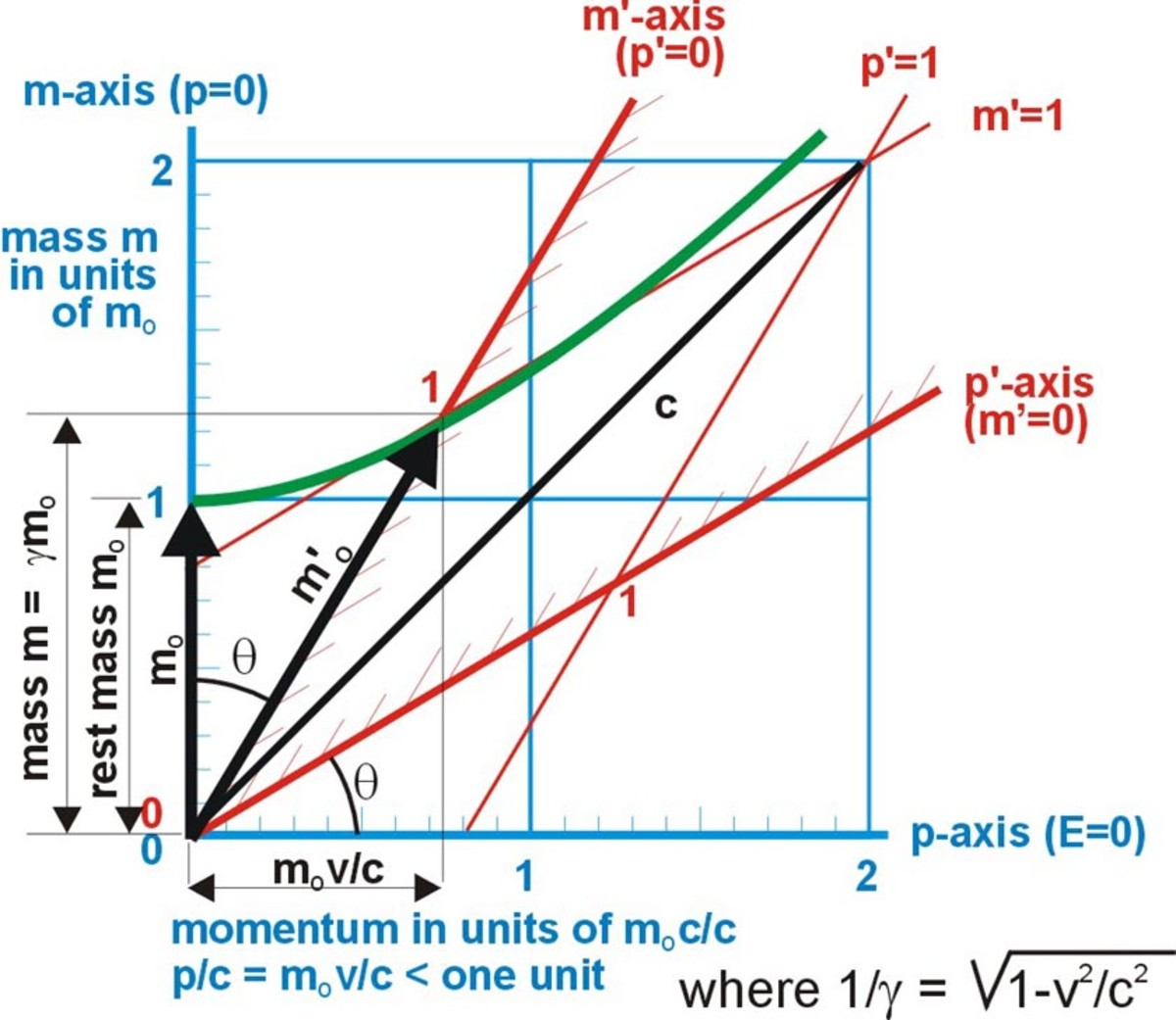Fig. 15 Lorentz transformations for mass and momentum at a relative velocity of 0.6c is (tan  = (p/c)/m = (mv/c)/m = v/c)

## Using the Minkowski diagram to show relationship between

In nonrelativistic physics, the kinetic energy (energy due to motion) of an object is K = ½mOv2. In relativistic physics the kinetic energy of an object is due to the increase in its mass due to its relative motion. Relativistic kinetic energy K = mc2 - mOc2. The rest energy of an object its rest mass times the speed of light squared

EO = mOc2. The total energy of an object is equal to its rest energy plus its kinetic energy. The total energy

E = EO + K = mc2 = gmOc2. In fig. 16 the magnitude of the total energy E = mc2 of the object particle plotted relative particle's momentum times c. We see that as the energy increases with pc. This increase follows the same hyperbola of invariance as the dilation of time.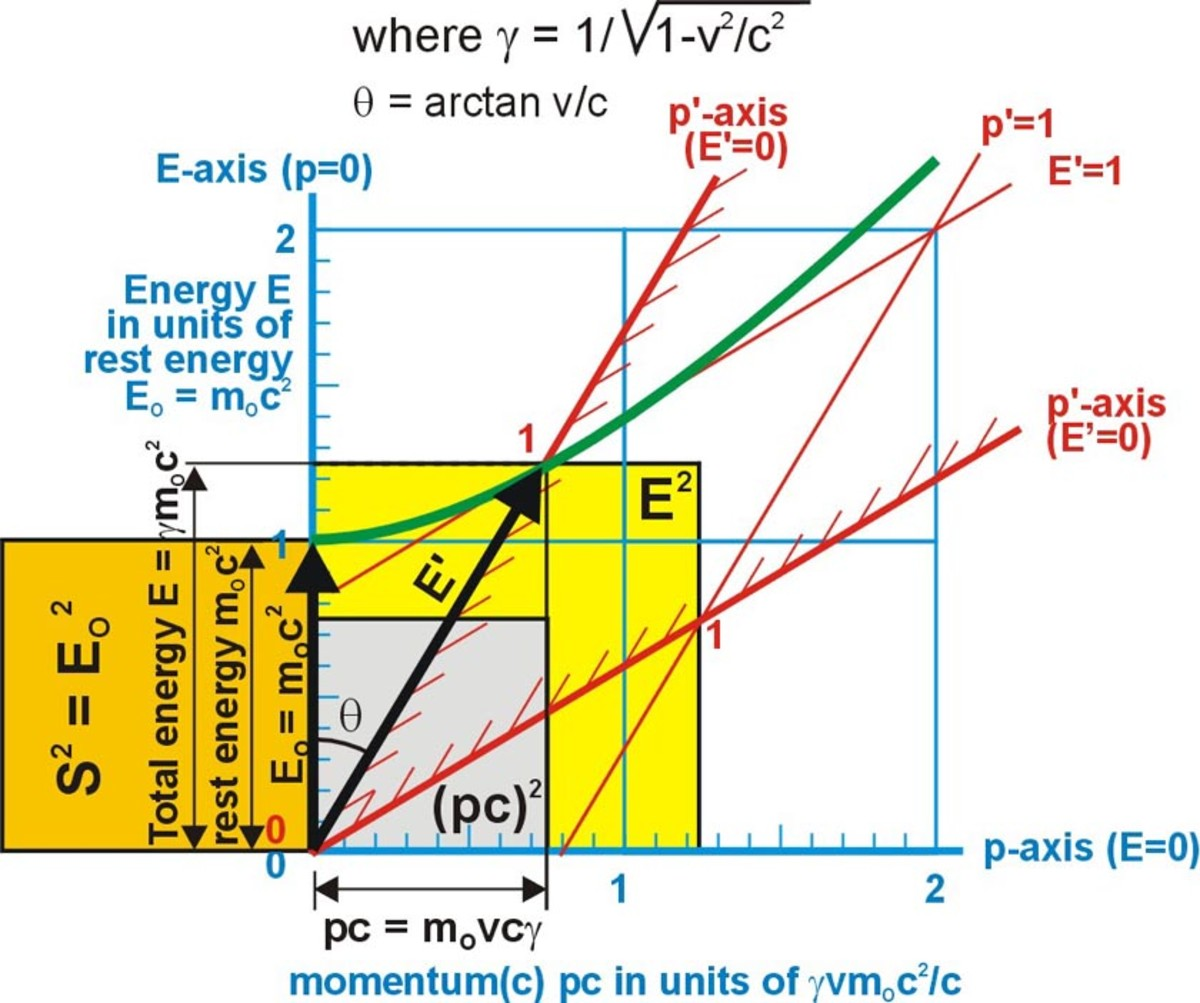Fig. 16 Lorentz transformations relating energy to momentum at a relative velocity of 0.6c (tan  = pc/EO = (mOvc)/mOc2 = v/c). Illustrated here as the EO2 = E2 - (pc) 2 = 12 = 1.252 – 0.752. The visible yellow area equals the orange area.

In fig 16 the total energy E = rest energy EO times g or EO cosh u. The momentum pc = mvc = gmOvc or

EO sinh u. The arrows EO and E'O illustrate the covariance of the energy since object's energy varies with the relative velocity. These same arrows illustrate the invariance of the rest energy since they both represent one unit of energy in each system. The EO arrow is one unit long in the observer's units. The E'O arrow is one unit long in the object's units.

*The momentum and energy relationship can be expressed by the equation E2 - (pc) 2 = Eo2. These equations can be illustrated on the diagram using the invariant S2 = [EO2 = E2 - (pc) 2 ] = [E'O2 = E'2 - (p'c) 2]. This diagram physical shows that relationship for S2, with the colored squares. The area of the orange square EO2 equals the area of the yellow square E2 minus the area of the gray square (pc) 2. The invariance is shown as a fundamental equation of a hyperbola, cosh2q -sinh2q = 1.

*Space-time Physics by Edwin F. Taylor & John A. Wheeler

## The invariance of like space-time areas and volumesFig. 17 Observer's viewpoint .........................Fig. 18 Object's viewpoint

## Comments

Steve on July 27, 2011:

Useful. Thanks.